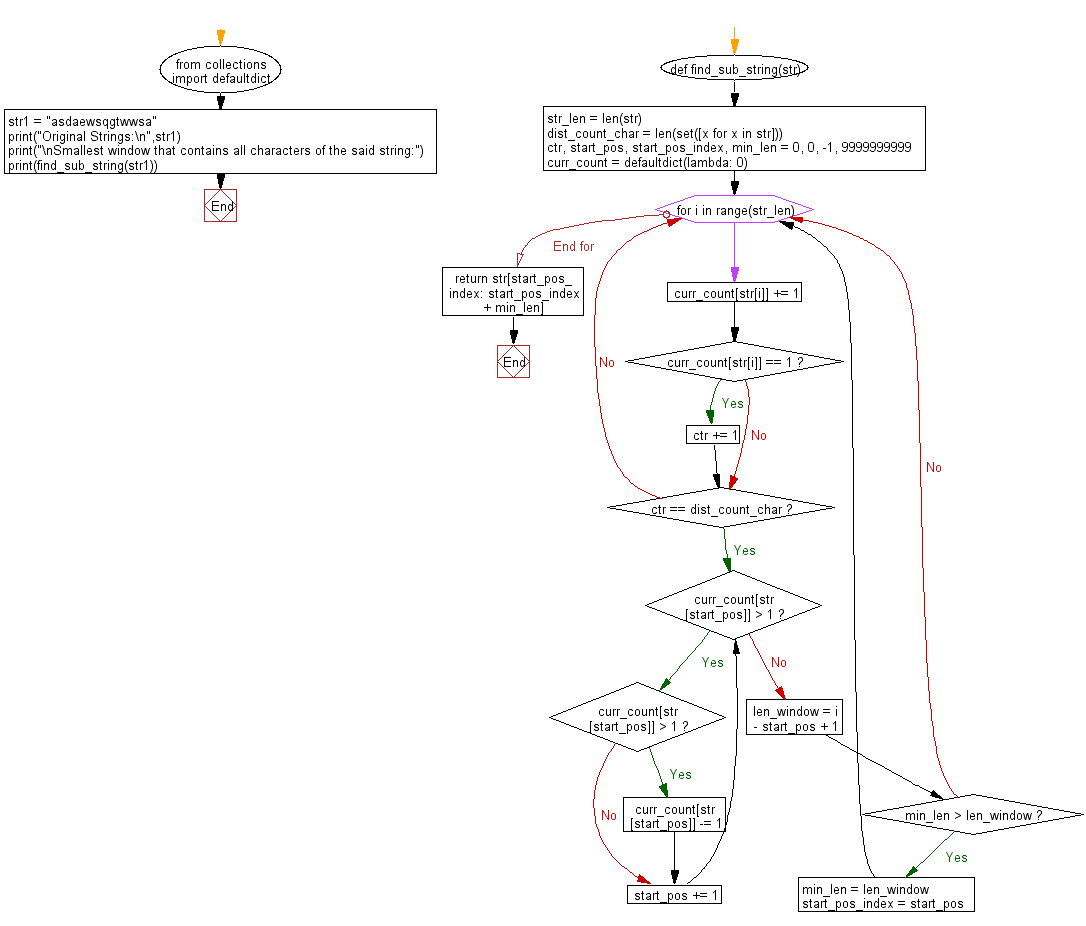﻿ Python: Find smallest window that contains all characters of a given string - w3resource# Python: Find smallest window that contains all characters of a given string

## Python String: Exercise-75 with Solution

Write a Python program to find smallest window that contains all characters of a given string.

Sample Solution:

Python Code:

``````from collections import defaultdict

def find_sub_string(str):
str_len = len(str)

# Count all distinct characters.
dist_count_char = len(set([x for x in str]))

ctr, start_pos, start_pos_index, min_len = 0, 0, -1, 9999999999
curr_count = defaultdict(lambda: 0)
for i in range(str_len):
curr_count[str[i]] += 1

if curr_count[str[i]] == 1:
ctr += 1

if ctr == dist_count_char:
while curr_count[str[start_pos]] > 1:
if curr_count[str[start_pos]] > 1:
curr_count[str[start_pos]] -= 1
start_pos += 1

len_window = i - start_pos + 1
if min_len > len_window:
min_len = len_window
start_pos_index = start_pos
return str[start_pos_index: start_pos_index + min_len]

str1 = "asdaewsqgtwwsa"
print("Original Strings:\n",str1)
print("\nSmallest window that contains all characters of the said string:")
print(find_sub_string(str1))
```
```

Sample Output:

```Original Strings:
asdaewsqgtwwsa

Smallest window that contains all characters of the said string:
daewsqgt
```

Pictorial Presentation:Flowchart:## Visualize Python code execution:

The following tool visualize what the computer is doing step-by-step as it executes the said program:

Python Code Editor:

Have another way to solve this solution? Contribute your code (and comments) through Disqus.

What is the difficulty level of this exercise?

Test your Programming skills with w3resource's quiz.

﻿

## Python: Tips of the Day

What is the difference between Python's list methods append and extend?

append: Appends object at the end.

```x = [1, 2, 3]
x.append([4, 5])
print (x)
```

Output:

```[1, 2, 3, [4, 5]]
```

extend: Extends list by appending elements from the iterable.

```x = [1, 2, 3]
x.extend([4, 5])
print (x)
```

Output:

```[1, 2, 3, 4, 5]
```

Ref: https://bit.ly/2AZ6ZFq